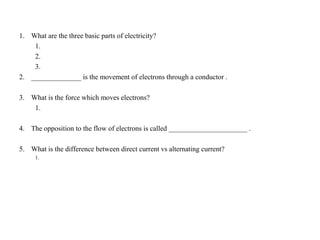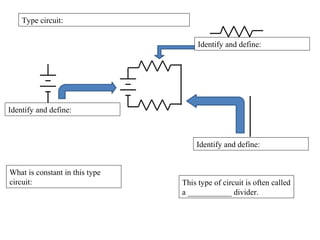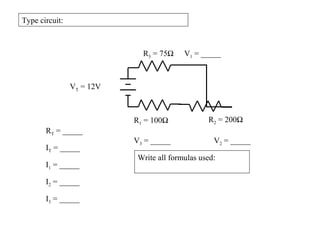Ce diaporama a bien été signalé.
Le téléchargement de votre SlideShare est en cours. ×Chargement dans…3
×

1 sur 10
1 sur 10

# Circuits 1

### Circuits 1

1. 1. 1. What are the three basic parts of electricity? 1. 2. 3. 2. ______________ is the movement of electrons through a conductor . 3. What is the force which moves electrons? 1. 4. The opposition to the flow of electrons is called ______________________ . 5. What is the difference between direct current vs alternating current? 1.
2. 2. Type circuit: Identify and define: Identify and define: Identify and define: What is constant in this type circuit: This type of circuit is often called a ___________ divider.
3. 3. Type circuit: R1 = 100Ω R2 = 200Ω R3 = 75Ω VT = 12V RT = _____ I1 = _____ I2 = _____ I3 = _____ IT = _____ Write all formulas used: V1 = _____ V2 = _____V3 = _____
4. 4. Type circuit: R1 = 1.5KΩ R2 = 2KΩ R3 = 1KΩ VT = 12V RT = _____ I1 = _____ I2 = _____ I3 = _____ IT = _____ Write all formulas used: V1 = _____ V2 = _____ V3 = _____
5. 5. Type circuit: R1 = 1KΩ R2 = 2KΩ R3 = 800Ω VT = ______ RT = _____ I1 = _____ I2 = _____ I3 = .00208A IT = _____ R4 = 1KΩ V1 = 2.08V V2 = _____ V3 = _____
6. 6. Type circuit: What is constant in this type circuit: This type of circuit is often called a ___________ divider.
7. 7. Type circuit: RT = _____ I1 = _____ I2 = _____ I3 = _____ IT = _____ V1 = _____ V2 = _____ V3 = _____ R1 = 1KΩ R2 = 2KΩ R3 = 800ΩVT = 12V
8. 8. Type circuit: RT = _____ I1 = _____ I2 = _____ I3 = _____ IT = _____ V1 = _____ V2 = _____ V3 = _____ R1 = 600Ω R2 = 1KΩ R3 = 800Ω VT = 12V
9. 9. The total resistance for a purely parallel circuit can be found by using the reciprocal formula RT = 1 1 + 1 + 1 R R R The total resistance for a purely series circuit can be found by using summation formula RT = R1 + R2 + R3
10. 10. R1 = _____ R2 = _____ R3 = _____ R4 = _____ R5 = _____ R6 = _____ R7 = _____ RT = _____ REq = _____ V1 = _____ V2 = _____ V3 = _____ V4 = _____ V5 = _____ V6 = _____ V7 = _____ I1 = _____ I2 = _____ I3 = _____ I4 = _____ I5 = _____ I6 = _____ I7 = _____ VT = _____ IT = _____